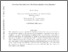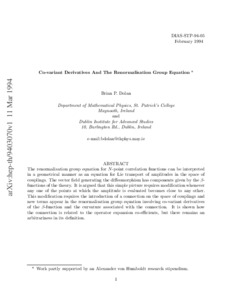# Co-variant Derivatives And The Renormalisation Group Equation

Dolan, Brian P. (1995) Co-variant Derivatives And The Renormalisation Group Equation. Working Paper. arXiv.Previewmore...Add this article to your Mendeley library

## Abstract

The renormalisation group equation for N-point correlation functions can be interpreted in a geometrical manner as an equation for Lie transport of amplitudes in the space of couplings. The vector field generating the diffeomorphism has components given by the β-functions of the theory. It is argued that this simple picture requires modification whenever any one of the points at which the amplitude is evaluated becomes close to any other. This modification requires the introduction of a connection on the space of couplings and new terms appear in the renormalisation group equation involving co-variant derivatives of the β-function and the curvature associated with the connection. It is shown how the connection is related to the operator expansion co-efficients, but there remains an arbitrariness in its definition.

Item Type: Monograph (Working Paper) Co-variant Derivatives; Renormalisation Group Equation; Faculty of Science and Engineering > Mathematical Physics 10507 https://doi.org/10.1142/S0217751X95001170 Dr. Brian Dolan 19 Feb 2019 15:21 arXivItem control page